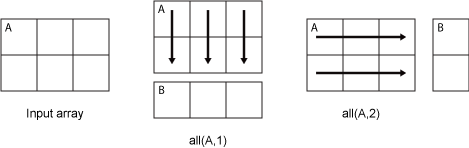# all

## 语法

``B = all(A)``
``B = all(A,'all')``
``B = all(A,dim)``
``B = all(A,vecdim)``

## 说明

````B = all(A)` 沿着大小不等于 1 的数组 `A` 的第一维测试所有元素为非零还是逻辑值 `1` (`true`)。实际上，`all` 是逻辑 AND 运算符的原生扩展。 如果 `A` 为向量，当所有元素为非零时，`all(A)` 返回逻辑 `1` (`true`)，当一个或多个元素为零时，返回逻辑 `0` (`false`)。如果 `A` 为非空矩阵，`all(A)` 将 `A` 的各列视为向量，返回包含逻辑 `1` 和 `0` 的行向量。如果 `A` 为 0×0 空矩阵，`all(A)` 返回逻辑 `1` (`true`)。如果 `A` 为多维数组，则 `all(A)` 沿第一个非单一维度运算并返回逻辑值数组。此维的大小将变为 `1`，而所有其他维的大小保持不变。 ```
``B = all(A,'all')` 对 `A` 的所有元素进行测试。此语法适用于 MATLAB® R2018b 及更高版本。`

``B = all(A,dim)` 沿着 `dim` 维测试元素。`dim` 输入是一个正整数标量。`

``B = all(A,vecdim)` 根据向量 `vecdim` 中指定的维度测试元素。例如，如果 `A` 是矩阵，则 `all(A,[1 2])` 对 `A` 中的所有元素进行测试，因为矩阵的每个元素均包含在由维度 1 和 2 定义的数组切片中。`

## 示例

`A = [0 0 3;0 0 3;0 0 3]`
```A = 3×3 0 0 3 0 0 3 0 0 3 ```
`B = all(A)`
```B = 1x3 logical array 0 0 1 ```

```A = [0.53 0.67 0.01 0.38 0.07 0.42 0.69]; B = (A < 0.5)```
```B = 1x7 logical array 0 0 1 1 1 1 0 ```

` if all(A < 0.5)`

` %do something`

` else`

` %do something else`

` end `

```A = rand(3,7,5) * 5; B = all(A(:) < 3)```
```B = logical 0 ```

`B = all(A(:) > 0)`
```B = logical 1 ```

`A(:)` 语法将 `A` 的元素转换成单列向量，因此您可以将此类型语句用于任意大小的数组。

`A = [0 0 3;0 0 3;0 0 3]`
```A = 3×3 0 0 3 0 0 3 0 0 3 ```

`B = all(A,2)`
```B = 3x1 logical array 0 0 0 ```

```A(:,:,1) = [2 1; 3 5]; A(:,:,2) = [0 0; 0 0]; A(:,:,3) = [-2 9; 4 1]; B = all(A,[1 2])```
```B = 1x1x3 logical array B(:,:,1) = 1 B(:,:,2) = 0 B(:,:,3) = 1 ```

## 输入参数

• `all(A,1)``A` 中列的连续元素进行处理并返回逻辑值行向量。

• `all(A,2)``A` 中行的连续元素进行处理并返回逻辑值列向量。